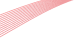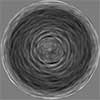# Strehl ratio as the Fourier transform of a probability density of error differences

Miguel A. Alonso and G. W. Forbes
in Optics Letters, Vol. 41, Issue 16, pp. 3735-3738 (2016).To give useful insight into the impact of mid-spatial frequency structure on optical performance, the Strehl ratio is shown to correspond to the Fourier transform of a simple statistical characterization of the aberration in the exit pupil. This statistical description is found simply by autocorrelating a histogram of the aberration values. In practice, the histogram itself can often be approximated by a convolution of underlying histograms associated with fabrication steps and, together with the final autocorrelation, it follows from the central limit theorem that the Strehl ratio as a function of the scale of the phase error is generally approximated well by a Gaussian.